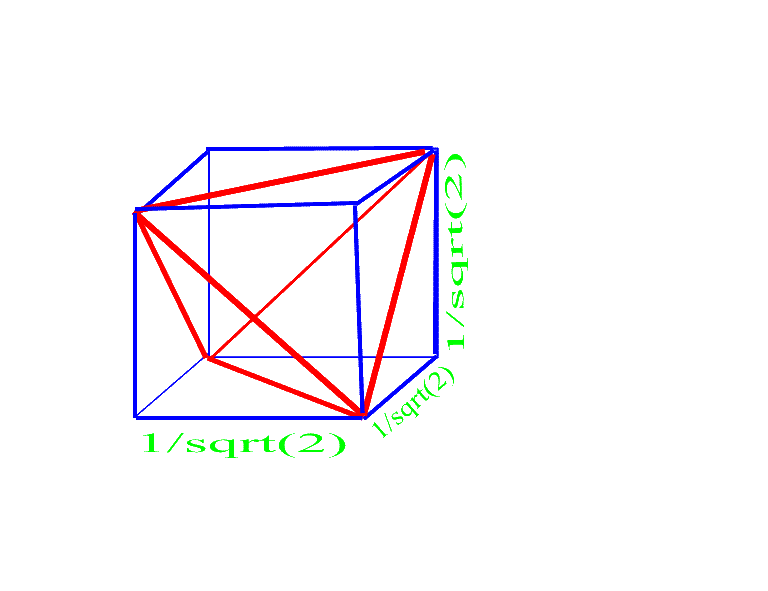# Problem 7 Solution

## Solution 1

The following can be derived using the Pythagorean theorem:

The height of a face is 3.5/2.

The area of any face is 3.5/4.

The distance from any corner to the center of a joining face is 1/3.5.

The height of the tetrahedron is (2/3).5

The area is 1/3*base*height =

(1/3) * 3.5/4 * (2/3).5 = 21/2/12.

## Solution 2

Provided by
Mircea Petrache, of "Liceo scientifico Varano" in Camerino, ItalyThe above cube has sides of length 1/sqr(2). The area formed by the diagonals along four of the faces as shown in the diagram is a tetrahedrom. Left over are four pyramids. The base of each pyramid is half of the side of the cube and the height is the height of the cube. Thus the area of each pyramid is: 1/2*(1/sqr(2))2 * 1/sqr(2) * 1/3 = (12*sqr(2))-1

The total area of the cube is (1/sqr(2))3 = (2*sqr(2))-1

The area of the tetrahedrom is thus (2*sqr(2))-1 - 4*(12*sqr(2))-1 =

3/(6*sqr(2)) - 2/(6*sqr(2)) = 1/(6*sqr(2)) = sqr(2)/12

Michael Shackleford, A.S.A.